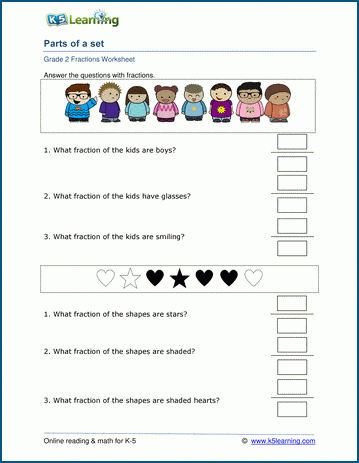# Fractions of a set

## Worksheets: Using fractions to describe a set

In these grade 2 fractions worksheets students are asked several questions about a group (or set) of objects.  Students answer the questions by writing fractions in standard form## More fractions worksheets

Explore all of our fractions worksheets, from dividing shapes into "equal parts" to multiplying and dividing improper fractions and mixed numbers.

## What is K5?

K5 Learning offers reading and math worksheets, workbooks and an online reading and math program for kids in kindergarten to grade 5.  We help your children build good study habits and excel in school.